NEET  >  Test: Fluid Mechanics - 1

# Test: Fluid Mechanics - 1

Test Description

## 30 Questions MCQ Test Physics Class 11 | Test: Fluid Mechanics - 1

Test: Fluid Mechanics - 1 for NEET 2023 is part of Physics Class 11 preparation. The Test: Fluid Mechanics - 1 questions and answers have been prepared according to the NEET exam syllabus.The Test: Fluid Mechanics - 1 MCQs are made for NEET 2023 Exam. Find important definitions, questions, notes, meanings, examples, exercises, MCQs and online tests for Test: Fluid Mechanics - 1 below.
Solutions of Test: Fluid Mechanics - 1 questions in English are available as part of our Physics Class 11 for NEET & Test: Fluid Mechanics - 1 solutions in Hindi for Physics Class 11 course. Download more important topics, notes, lectures and mock test series for NEET Exam by signing up for free. Attempt Test: Fluid Mechanics - 1 | 30 questions in 60 minutes | Mock test for NEET preparation | Free important questions MCQ to study Physics Class 11 for NEET Exam | Download free PDF with solutions
 1 Crore+ students have signed up on EduRev. Have you?
Test: Fluid Mechanics - 1 - Question 1

### A bucket contains water filled upto a height = 15 cm. The bucket is tied to a rope which is passed over a frictionless light pulley and the other end of the rope is tied to a weight of mass which is half of that of the (bucket + water). The water pressure above atmosphere pressure at the bottom is :

Detailed Solution for Test: Fluid Mechanics - 1 - Question 1

Consider the mass of the block be m, thus we get a mass of bucket + water is 2m. Now if we consider it as a two mass pulley, we get  the net acceleration a by which the bucket is going down can be found by -
2mg - mg = 3ma
Thus we get a = g/3
Now we know that the bucket is accelerating with acceleration g/3 downwards hence to treat it as an inertial system we need to apply pseudo force upon it in upward direction. Thus we get in reference to the bucket net acceleration is 2g/3 downwards. Thus the pressure at the bottom is P + x 0.15 x 2g/3 = P + 1 kPa

Test: Fluid Mechanics - 1 - Question 2

### A uniformly tapering vessel shown in Fig. is filled with liquid of density 900 kg/m3. The force that acts on the base of the vessel due to liquid is (take  g = 10 m/s2) -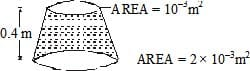Test: Fluid Mechanics - 1 - Question 3

### A liquid of mass 1 kg is filled in a flask as shown in figure. The force exerted by the flask on the liquid is (g = 10 m/s2) [Neglect atmospheric pressure]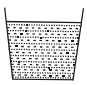Detailed Solution for Test: Fluid Mechanics - 1 - Question 3

The force exerted by the flas on the liquid is equal to the force applied by the liquid on the flask which is equal to its weight = mg = 10N

Test: Fluid Mechanics - 1 - Question 4

A U-tube having horizontal arm of length 20 cm, has uniform cross-sectional area = 1 cm2. It is filled with water of volume 60 cc. What volume of a liquid of density 4 g/cc should be poured from one side into the U-tube so that no water is left in the horizontal arm of the tube?

Detailed Solution for Test: Fluid Mechanics - 1 - Question 4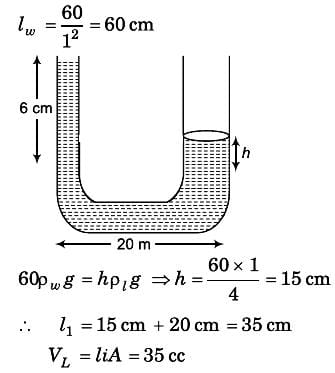Test: Fluid Mechanics - 1 - Question 5

A light semi cylindrical gate of radius R is piovted at its mid point O, of the diameter as shown in the figure holding liquid of density r. The force F required to prevent the rotation of the gate is equal to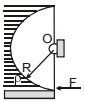Detailed Solution for Test: Fluid Mechanics - 1 - Question 5

The force is normal to the surface of the cylinder and hence will pass through the centre. And since the forces pass through the centre it means that the net torque will be zero.

Test: Fluid Mechanics - 1 - Question 6

The pressure at the bottom of a tank of water is 3P where P is the atmospheric pressure. If the water is drawn out till the level of water is lowered by one fifth., the pressure at the bottom of the tank will now be

Test: Fluid Mechanics - 1 - Question 7

An open-ended U-tube of uniform cross-sectional area contains water (density 1.0 gram/centimeter3) standing initially 20 centimeters from the bottom in each arm. An immiscible liquid of density 4.0 grams/centimeter3 is added to one arm until a layer 5 centimeters high forms, as shown in the figure above. What is the ratio h2/h1 of the heights of the liquid in the two arms ?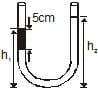Detailed Solution for Test: Fluid Mechanics - 1 - Question 7

5 × 4 × 10 + (h1 - 5) × 10 = h2 × 1 × 10
200 + 10h1 – 50 = 10h2
10h2 - 10h1 = 150
h2 - h1 = 15
h2 + h1 = 20 + 20 + 5 = 45
2h2 = 60
h2 = 30
h1 = 15
h2/h1 = 30/15
h2/h1 = 2/1

Test: Fluid Mechanics - 1 - Question 8

The area of cross-section of the wider tube shown in figure is 800 cm2. If a mass of 12 kg is placed on the massless piston, the difference in heights h in the level of water in the two tubes is :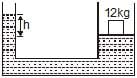Detailed Solution for Test: Fluid Mechanics - 1 - Question 8

h × ρ × 10 = 120/800 × 10-4
h × ρ = 12/8 × 10
h × ρ = 150m
h × 1000 = 150m
h = 15cm

Test: Fluid Mechanics - 1 - Question 9

A body is just floating in a liquid (their densities are equal) If the body is slightly pressed down and released it will -

Detailed Solution for Test: Fluid Mechanics - 1 - Question 9

The body will sink to the bottom as it gains a downward velocity and has no force to bring it back up. The weight becomes greater than upwards thrust.

Test: Fluid Mechanics - 1 - Question 10

Two stretched membranes of areas 2 and 3 m2 are placed in a liquid at the same depth. The ratio of the pressure on them is -

Detailed Solution for Test: Fluid Mechanics - 1 - Question 10

Pressure depends on depth of container irrespective of its shape and in the above case depth for both the vessels are given same. Therefore the hydraulic pressure for the containers will be in the ratio 1: 1

Test: Fluid Mechanics - 1 - Question 11

An ice block floats in a liquid whose density is less than water. A part of block is outside the liquid. When whole of ice has melted, the liquid level will -

Detailed Solution for Test: Fluid Mechanics - 1 - Question 11

Ice is lighter than water. When ice melts, the volume occupied by water is less than that of ice, due to which the level of water goes down.

Test: Fluid Mechanics - 1 - Question 12

A fluid container is containing a liquid of density r is is accelerating upward with acceleration a along the inclined place of inclination a as shwon. Then the angle of inclination q of free surface is :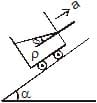Detailed Solution for Test: Fluid Mechanics - 1 - Question 12

First resolve all components in the along and perpendicular to incline. Pressure difference is created in a vertical column full of liquid. This is because of gravity acting in downward direction. Similarly, pressure difference will be created too along the incline. So, p = h * d * g * cosa (in perpendicular direction) and
p = hd (a + g sina) (along incline).
So, tan(theta) = (a + gsina)/(gcosa)

Test: Fluid Mechanics - 1 - Question 13

Figure shows a three arm tube in which a liquid is filled upto levels of height l. It is now rotated at an angular frequency w about an axis passing through arm B. The angular frequency w at which level of liquid of arm B becomes zero.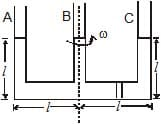Detailed Solution for Test: Fluid Mechanics - 1 - Question 13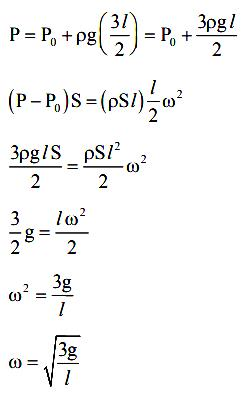Test: Fluid Mechanics - 1 - Question 14

Two cubes of size 1.0 m sides, one of relative density 0.60 and another of relative density = 1.15 are connected by weightless wire and placed in a large tank of water. Under equilibrium the lighter cube will project above the water surface to a height of

Detailed Solution for Test: Fluid Mechanics - 1 - Question 14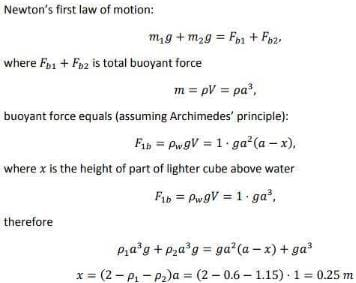which is equal to 25 cm.

Test: Fluid Mechanics - 1 - Question 15

A cuboidal piece of wood has dimensions a, b and c. Its relative density is d. It is floating in a larger body of water such that side a is vertical. It is pushed down a bit and released. The time period of SHM executed by it is :

Detailed Solution for Test: Fluid Mechanics - 1 - Question 15

Time period of SHM of small vertical oscillations in a liquid is given by T=2π√l/g, where l is the length of cube/cylinder dipped in the water.
So according to law of floatation,
weight of the cube = weight of the water displaced
abc × d × g=bcl × 1×g
⇒l=da
⇒T=2π√da/g

Test: Fluid Mechanics - 1 - Question 16

Two bodies having volumes V and 2V are suspended from the two arms of a common balance and they are found to balance each other. If larger body is immersed in oil (density d1 = 0.9 gm/cm3) and the smaller body is immersed in an unknown liquid, then the balance remain in equilibrium. The density of unknown liquid is given by :

Test: Fluid Mechanics - 1 - Question 17

A boy carries a fish in one hand and a bucket (not full) of water in the other hand. If the places the fish in the bucket, the weight now carried by him (assume that water does not spill) :

Detailed Solution for Test: Fluid Mechanics - 1 - Question 17

Buoyant force on fish due to water will be equal and opposite to the force on water by fish.
These two forces are internal forces for the fish bucket system.
Hence, they will not affect the weight the boy carries.

Test: Fluid Mechanics - 1 - Question 18

A piece of steel has a weight W in air, W1 when completely immersed in water and W2 when completely immersed in an unknown liquid. The relative density (specific gravity) of liquid is :

Detailed Solution for Test: Fluid Mechanics - 1 - Question 18

If the loss of weight of a body in water is 'a' while in liquid is 'b' then
Sigma in liquid / sigma in water = upthrust on body in liquid / upthrust on body in water
Then a / b = (W air - W liquid) / (W air - W water).

Test: Fluid Mechanics - 1 - Question 19

A ball of relative density 0.8 falls into water from a height of 2m. The depth to which the ball will sink is (neglect viscous forces) :

Detailed Solution for Test: Fluid Mechanics - 1 - Question 19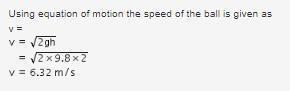Let us calculate the buoyancy force by water try to stop the ball.
Buoyancy force = weight of displaced water
= Density of water x Volume of the ball x g
= d x V x g  --- (1)
But buoyant force = ma
Therefore, ma = d x V x g
or a = (dVg) / m --- (2)
Let the density of the ball be d'.
→ m = d'V
Substituting in equation 2, we get
a = (dVg) / d'V
= dg / d'
= (d/d') x g
Given that relative density, (d / d') = 0.8
So, a = g / (0.8)
= 10 / 0.8
→ a = 12.5 m/s^2
Net deceleration of ball, a' = a - g = 12.5 - 10
= 2.5 m/s^2
Final speed of ball v' = 0
Using the equation - v'^2 = v^2 + 2a's (where s = depth of ball in the water)
Substituting the values in the above equation, we get
40 = 0 + 2 x 2.5 x s
s = 8m

Test: Fluid Mechanics - 1 - Question 20

A cube of iron whose sides are of length L, is put into mercury. The weight of iron cube is W. The density of iron is rI, that of mercury is rM. The depth to which the cube sinks is given by the expression _

Test: Fluid Mechanics - 1 - Question 21

A metal ball of density 7800 kg/m3 is suspected to have a large number of cavities. It weighs 9.8 kg when weighed directly on a balance and 1.5 kg less when immersed in water. The fraction by volume of the cavities in the metal ball is approximately :

Detailed Solution for Test: Fluid Mechanics - 1 - Question 21

Here when put in water the water displaces/fills the empty cavity.
Volume of only metal = mass/density = 9.8/7800 = 1.25X10-3 cubic m
Volume of whole ball including cavity = weight of water displaced/density of water
Density of water is 1000 kg/cubic m.
Volume of whole ball = 1.5X10-3 cubic m
Volume of cavity = Volume of ball - volume of metal
= 1.5X10-3 - 1.25X10-3
= 0.25X10-3 cubic m
Ratio of volume of cavity/ball = 0.25X10^-3 / 1.5X10^-3 = 0.16 = 16%
0.16 fraction of whole ball is a cavity i.e. 16%

Test: Fluid Mechanics - 1 - Question 22

A sphere of radius R and made of material of relative density s has a concentric cavity of radius r. It just floats when placed in a tank full of water. The value of the ratio R/r will be

Detailed Solution for Test: Fluid Mechanics - 1 - Question 22

If the sphere is "just" floating means the sphere is exactly immersed in water (or) the buoyant force acting on it is exactly equal to its weight. The cavity reduces the weight of the actual sphere but has no role in the buoyant force.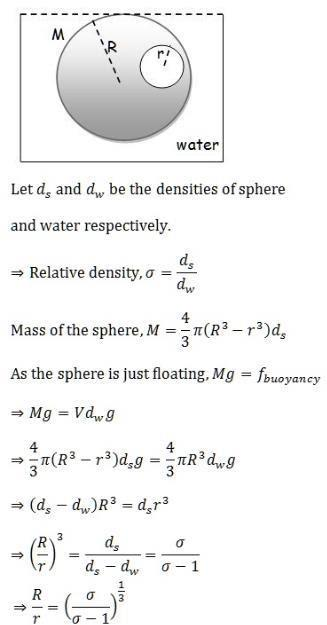Test: Fluid Mechanics - 1 - Question 23

A beaker containing water is placed on the platform of a spring balance. The balance reads 1.5 kg. A stone of mass 0.5 kg and density 500 kg/m3 is immersed in water without touching the walls of beaker. What will be the balance reading now ?

Detailed Solution for Test: Fluid Mechanics - 1 - Question 23

Since the weight of the block must be equal to the buoyant force acting on the block for it to remain in equilibrium,

B=0.5kg

The reading of the spring balance = Weight of water + Buoyant force' reaction pair force downwards

=1.5kg+0.5kg=2kg

Test: Fluid Mechanics - 1 - Question 24

A cylindrical block of area of cross-section A and of material of density r is placed in a liquid of density one-third of density of block. The block compresses a spring and compression in the spring is one-third of the length of the block. If acceleration due to gravity is g, the spring constant of the spring is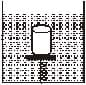Detailed Solution for Test: Fluid Mechanics - 1 - Question 24

Since the block is at rest, net force on the cylinderical block is zero, ie net upward = net downward force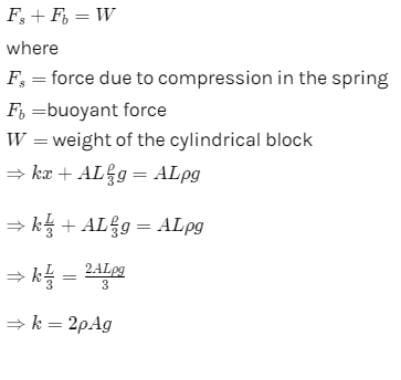Test: Fluid Mechanics - 1 - Question 25

A body of density r' is dropped from rest at a height h into a lake of density r, where r > r'. Neglecting all disipative froces, calculate the maximum depth to which the body sinks before returning of float on the surface.

Test: Fluid Mechanics - 1 - Question 26

Water is flowing in a horizontal pipe of

non-uniform cross - section. At the most contracted place of the pipe –

Detailed Solution for Test: Fluid Mechanics - 1 - Question 26

Continuity equation states that, "For a non-viscous liquid and streamlined flow the volume flow rate (Area of cross section x velocity) is constant throughout the flow at any point".
According to this, Av = constant. So if at any point the cross-section area decreases then velocity of liquid at that point increases and vice-versa.
Bernoulli's equation states that, "For a streamlined and non-viscous flow the total energy (kinetic energy and pressure gradient) remains constant throughout the liquid.
According to this, kinetic energy + Pressure gradient = constant. So, if at any point the velocity increases the pressure at that point decreases and vice-versa.
At the most contracted place of the pipe area of cross section is minimum
⇒ velocity is maximum
⇒ pressure is minimum

Test: Fluid Mechanics - 1 - Question 27

Water is flowing in a tube of non-uniform radius. The ratio of the radii at entrance and exit ends of tube is 3 : 2. The ratio of the velocities of water entering in and exiting from the tube will be –

Detailed Solution for Test: Fluid Mechanics - 1 - Question 27

We know, for the fluid flowing through the non-uniform pipe the velocity of fluid is inversely proportional to the area of cross-section.
Hence, if v1, v2 are the velocities of entry and exit end of the pipe and a1, a2 are the area of cross-sections of entry and exit end of the pipe, then
v1/v2=a2/a1
⇒v1/v2​=(r2)2/(r1)2
∴v1/v2​=(2)2/(3)2​=4/9​

Test: Fluid Mechanics - 1 - Question 28

A rectangular tank is placed on a horizontal ground and is filled with water to a height H above the base. A small hole is made on one vertical side at a depth D below the level of the water in the tank. The distance x from the bottom of the tank at which the water jet from the tank will hit the ground is

Detailed Solution for Test: Fluid Mechanics - 1 - Question 28

Time taken by liquid drop to fall
S=ut+(1/2)​at2
t=√2(H−D)/​​g
Horizontal velocity of liquid =√2gD​
Range =√2(H−D)/g​​× √2gD​
Range =2√D(H−D)

Test: Fluid Mechanics - 1 - Question 29

A jet of water with cross section of 6 cm2 strikes a wall at an angle of 60º to the normal and rebounds elastically from the wall without losing energy. If the velocity of the water in the jet is 12 m/s, the force acting on the wall is

Detailed Solution for Test: Fluid Mechanics - 1 - Question 29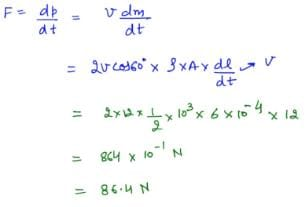Test: Fluid Mechanics - 1 - Question 30

The cross sectional area of a horizontal tube increases along its length linearly, as we move in the direction of flow. The variation of pressure, as we move along its length in the direction of flow (x-direction), is best depicted by which of the following graphs

Detailed Solution for Test: Fluid Mechanics - 1 - Question 30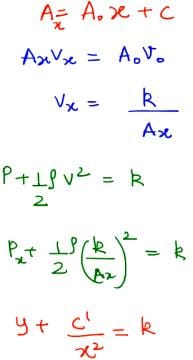So, it will be a downward facing parabola.

## Physics Class 11

127 videos|464 docs|210 tests
 Use Code STAYHOME200 and get INR 200 additional OFF Use Coupon Code
Information about Test: Fluid Mechanics - 1 Page
In this test you can find the Exam questions for Test: Fluid Mechanics - 1 solved & explained in the simplest way possible. Besides giving Questions and answers for Test: Fluid Mechanics - 1, EduRev gives you an ample number of Online tests for practice

## Physics Class 11

127 videos|464 docs|210 tests

### How to Prepare for NEET

Read our guide to prepare for NEET which is created by Toppers & the best Teachers